Main Page > SSD projects > Large Scale Numerical Simulation Project

### Large Scale Numerical Simulation Project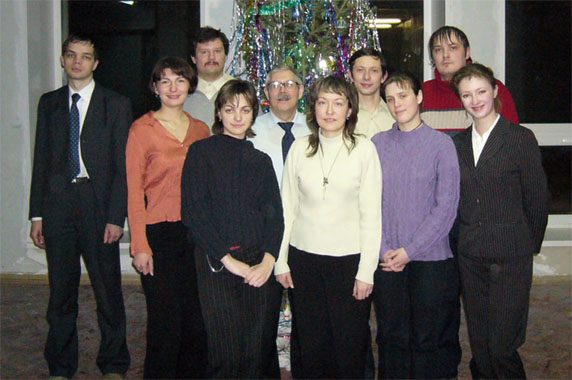Research group photo

### Participants:

Abstract:
With MVS-1000M the distribution of particle and gas density in the soliton were investigated, as well as their velocities, angular momentum, flow of gas and particles through soliton and vorticity of particle velocity. It is shown that in the soliton the high values of gas and dust densities and gas pressure arise. This fact probably explains the synthesis of organic compounds in protoplanetary disc.

Introduction:
In recent times there is a great interest to the problem of organic matter genesis in the solar System. In \cite{Gleaves} the protoplanetary disc is considered as a catalytic chemical reactor for synthesis of primary organic compounds. The mean density and temperature in protoplanetary disc are very small. Because of this reason it is extremely important to find out how do the high values of temperature, pressure and density appear, that are necessary for chemical synthesis. The solitons that appeared in our computational experiments present one of the possible answers to this question.

Source equations
The dynamics of the dust component of protoplanetary disc is described by the Vlasov-Liouville kinetic equation. In the following text dust particles will be called simply particles. To consider motion of the gas component the equations of gas dynamics are employed. The gravitational field is determined by Poisson equation. If we employ the collisionless approximation of the mean self-consistent field, then Vlasov-Liouville kinetic equation is written in the following form: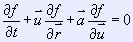where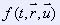is is the time-dependent one-particle distribution function along coordinates and velocities,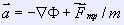is the acceleration of unit mass particle,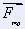is the friction force between gas and dust components of the medium. Gravitational potential,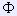, could be divided
into two parts: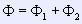where1 presents either the potential of immobile central mass (either galactic black hole or protostar) or the potential of a rigid system which is out of disc plane (either the stars of galactic halo or molecular cloud). The second part of potential2 is determined by the additive distribution of the moving particles and gas.2 satisfies the Poisson equation: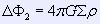In the case of infinitesimally thin disc the bulk density of the mobile media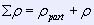is equal to zero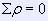(part is the particle density,is the gas density). At the disc with the surface density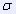.This shear gives a boundary condition for the normal derivative of potential2: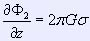The equations of gas dynamics take the following form: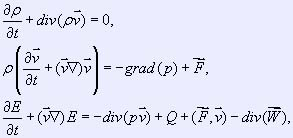where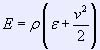is the density of gas full energy,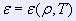is the internal energy, p = p(,T) is the gas pressure, W is the heat flow, is the increase of energy due to chemical reactions and radiation. F is the external force which is defined by the following expression: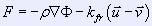where kfr is the coefficient of friction between gas and dust components of the disc, u is the dust velocity, v is the gas velocity.
In the case of the flat disc (so called 3D2V model) the form of equation remains the same with the only exclusion: bulk densityis replaced with surface density.In this paper, we shall consider only the flat disc model.

In the full description of the protoplanetary disc these equations are complemented with the equations for chemical reactions in gas phase and the equations for simulation of coagulation processes in the dust component.
The following quantities were chosen as basic characteristic parameters for transition to sizeless variables:

– distance from the Sun to the Earth R0= 1.5* 1011m;
– mass of the Sun M0= 2* 1030kg;
– gravitational constant G= 6.67* 10-11m2* kg-2.

Corresponding characteristic values of the particle velocity V0, time t0, potential0, density0(0) and pressure p0 are written as: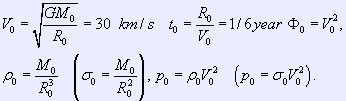In the following text all the parameters are given in sizeless units.
Vlasov-Liouville equation is solved by Particles-in-Cells method . To solve the equations of gas dynamics Fluids-in-Cells method is employed .
In the authors' opinion, an efficient Poisson equation solver could be built only if the peculiarities of the problem were taken into account. These peculiarities are the following. First, the problem is non-stationary. Second, at initial moment of time the disc has axial symmetry. And third, all the matter is situated in the disc plain. Having considered all these peculiarities, a special Poisson equation solver was built on the basis of fast Fourier transform .
The implemented methods for solution of Vlasov equation and gas dynamics equations are described in more detail in . Parallelisation scheme is presented in .
Initial distribution of the particle and gas density is set according to the model of solid body rotation: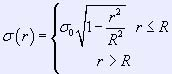Here R is the radius of the corresponding disc. Initially for radial component of the particles' velocities a normal distribution is set: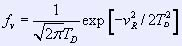here TD is the dynamic temperature of particles. An additional parameter is the pressure of gas in the disc centre.

Parameters of computational experiments:
The technical parameters of computational experiments are given in the table 1

 Number of grid nodes NR 120 200 300 NR 128 256 256 Nz 128 128 128 Number of particles (in millions) 10 20 50 Computation time (in hours) 8 30 49 Number of processors 8.0 Radius of domain 9.0 Height of domain 8.0 Table 1. Technical parameters.

The physical parameters that are set as initial data for the computational experiment are given in the table 2. The most important among them are the masses: mass of central body, mass of gas component and mass of dust component

 Radius of dust disc, RP 1.0 Radius of gas disc, RG 1.0 Mass of dust component, MP 1.0 Mass of gas component, MG 1.0 Mass of central body, M0 0.5 Dynamic temperature of dust particles, TD 1.0 Coefficient of friction, kfr 1.0 Gas pressure in the centre, p0 0.01 Table 2. Physical parameters

In the course of computational experiments it was found out that the solitons definitely arise when the mass ratio is MP : MG : M0 = 2 : 2 : 1. Also soliton formation is affected by the coefficient of friction between dust and gas components. When this coefficient substantionally decreases the solitons do not arise. The increase of the radius of either gas or dust component affects the system like the decrease of the corresponding mass and makes the disc more unstable. The dynamic temperature of dust particles and gas pressure in the disc centre make the corresponding component of the disc more stable. Nevertheless the influence of these quantities on the soliton formation is a question of further studies.
Generally about 40 soliton simulation experiments were conducted with MVS-1000M. Six computational experiments of this array are presented in this paper. The physical parameters were identical in all experiments, but the number of particles and grid size were different. From the table 1 it follows that such experiments require large computatational resources. To compute dust and gas motion even with a small grid it is necessary to employ 8 processors. The computational experiments on finer grids were conducted with the so called "checkpoints". A checkpoint means that all the data of the program are saved to a file, one file for each processor, and then the program stops. After a while, the computational experiment is continued starting from the saved data.

 Grid size Number of particles Size of region under study Center of region under study I 120*128*128 10 million 2.0*2.0 (0.0, 0.0) II 200*256*128 25 million 2.0*2.0 (0.0, 0.0) III 200*256*128 25 million 0.3*0.3 (0.6, 0.6) IV 200*256*128 25 million 0.4*0.4 (-0.71, 0.1) V 300*256*128 50 million 3.0*3.0 (0.0, 0.0) VI 300*256*128 50 million 1.0*1.0 (0.5, 0.5) Table 3.Computational experiments presented in the paper.

The difference between the computational experiments is shown in the table 3. The computations are conducted with the grid in cylindrical coordinate system. Nevertheless, to draw a 2D spatial distribution of some function h(r,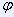) a Cartesian grid is employed. This Cartesian grid is introduced in the same domain and the distribution - now h(x,y) is computed on this grid directly with particles.
Moreover, a small region of the disc is selected for detailed study, and in this region the distribution h(x,y) is drawn with a Cartesian grid of the same size as in the whole disc. The same way a telescope shows some part of the sky in more detail. In the computational experiments with the same grid size and the same number of particles different regions of the disc where considered. From both the physical and the computational point of view it is the same experiment. Nevertheless, it is necessary to conduct a number of experiments with the same parameters due to the following reasons. First, it is impossible to draw the distribution in the whole disc in great detail. Second, it becomes clear which region of the disc is worth studying in detail only when the experiment is over. It should be noticed that there is no loss of precision, since the distribution in a small part of the disc is also computed with particles.
The pictures in the section Soliton Structure are an exclusion from this rule. They present distributions from the vicinity of the soliton, that is, in a region of a very small size (less than 0.2 * 0.2). They are obtained by cutting the corresponding part of the grid from the distribution in a large region.
Both in the whole disc and in small size regions the distribution is drawn by the same rules: white color corresponds to maximal value, black to zero, the scale is logarithmic.

Grid influence on the computational experiments.
Computational experiments with the same physical parameters were conducted on the following sequence of grids: 120*128*128 nodes, 200*256*128 nodes, 300*256*128 nodes. The resulting distributions of particle density are shown in fig. 1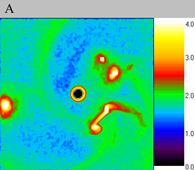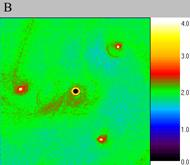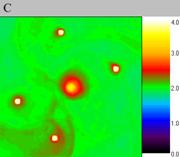Fig. 1. Density waves on different grids.

The size of the exposed domain is 2.0*2.0, coordinates of the centre are (0.0, 0.0), moment of time is 4.0. A – 120*128*128 nodes (computational experiment I), B - 200*256*128 nodes (computational experiment II), C - 300*256*128 nodes(computational experiment V).
Density waves that arise on the coarse grid (120?128?128 nodes) are different from solitons in that they have large size, as it is seen in fig. 1. A in comparison with figures 1B and 1C. Another difference is that they are unstable. These density waves decay in a short period of time "delta" T = 0.4, fig. 2C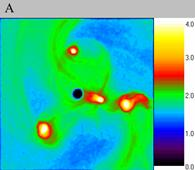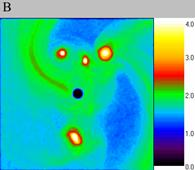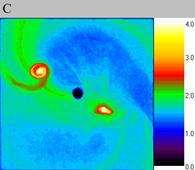Fig. 2. Decay of density waves on the coarse grid, computational experiment I. The size of the exposed domain is 2.0*2.0, the centre of it is in (0.0, 0.0). Figure A shows particle density distribution for the moment of time 4.4, figure B for the moment of time 4.8 and figure C for 5.2.

On the contrary, on finer grids density waves have small size (fig. 3) and they remain stable during a large period of time, ?T = 4.0, figure 3, A, B and C. In fig. 3C it is seen that upper and lower solitons are shifted from initial position. But the most important result is that these density waves remain stable and keep small size.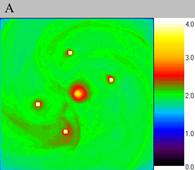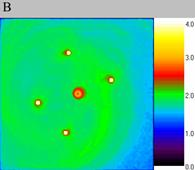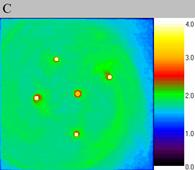Fig. 3.The stability of density waves on the fine grid, computational experiment V. The size of the exposed domain is 3.0*3.0, the centre of it is in (0.0, 0.0). Figure A shows particle density distribution for the moment of time 4.0, figure B for the moment of time 6.0 and figure C for 8.0.

Soliton behavior:
Soliton is a lone density wave. On the fine grid (computational experiment V) the solitons were nearly immobile during a short period of time. Considered particle density distribution in dynamics it is clearly seen. In fig. 4 this distribution is shown in the three successive moments of time. The difference in time between them is 0.2. The four solitons do not shift during this short period, though the density distribution in the whole disc changes significantly.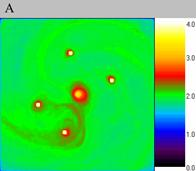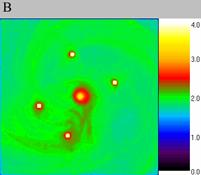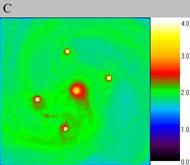Fig. 4.The immobile solitons on the fine grid, computational experiment V. The size of the exposed domain is 3.0?3.0, the centre of it is in (0.0, 0.0). Figure A shows particle density distribution for the moment of time 4.0, figure B for the moment of time 4.2 and figure C for 4.4.

The following fact proves that these structures are density waves and not clumps of dust. The matter of the disc rotates around the disc centre, but the solitons remain in its position. It should be noticed that in fact the soliton moves as a density wave, but its velocity could be oriented either along the flow or in the opposite direction.
So the soliton form a small region of high density in the flow. It is similar to a magnetic lens that focuses a beam of charged particles. The difference is that the focusing field in the case of soliton is created by the beam itself.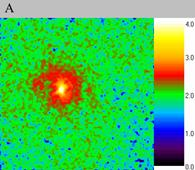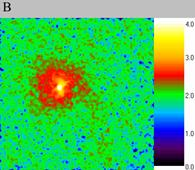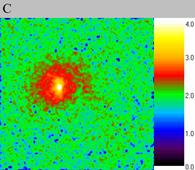Fig. 5.The immobile soliton scaled-up, computational experiment III. The size of the exposed domain is 0.3*0.3, the centre of it is in (0.6, 0.6). Figure A shows particle density distribution for the moment of time 4.01, figure B for the moment of time 4.05 and figure C for 4.09.

Let us consider the soliton scaled-up. With the same physical parameters the soliton either remained nearly immobile (computational experiment III, fig. 5) or oscillated around some point (computational experiment IV, fig. 6). Let us remind that these are two different solitons from the same computational experiment.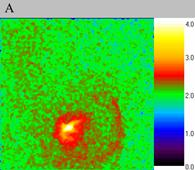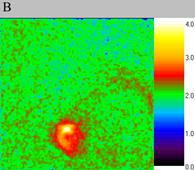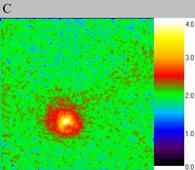Fig. 6.. The oscillating soliton scaled-up, computational experiment IV. The size of the exposed domain is 0.4?0.4, the centre of it is in (-0.71, 0.1). Figure A shows particle density distribution for the moment of time 4.01, figure B for the moment of time 4.09 and figure C for 4.17.

In the computational experiment VI the absorption of a density wave by soliton occured, fig. 7. First the density wave arises (fig. 7A), then it approaches the soliton (fig. 7B). After absorption the soliton deviates from the initial position (fig. 7C) but then returns back (fig. 7D). It should be noticed that such a phenomenon is impossible for clumps. Thus figure 7 proves wave nature of the structures observed in our computational experiments - the solitons.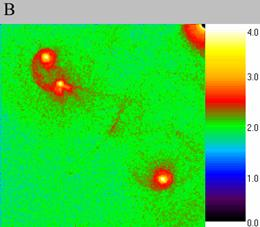T = 4.76 T = 4.96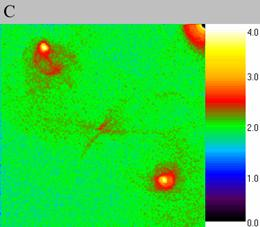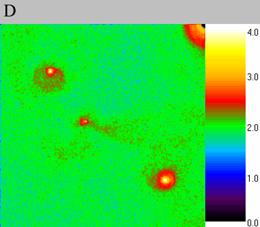T = 5.04 T = 5.2 Fig. 7..Absorption of a density wave by the soliton, computational experiment VI. The size of the exposed domain is 1.0*1.0, the centre of it is in (-0.5, -0.5). Figure A shows particle density distribution for the moment of time 4.76, figure B for the moment of time 4.96, figure C for 5.04 and figure D for 5.2.

### Literature:

1. Snytnikov V.N., Dudnikova G.I., Gleaves J.T., Nikitin S.A., Parmon V.N., Stoyanovsky V.O., Vshivkov V.A., Yablonsky G.S.,Zakharenko V.S.. Space chemical reactor of protoplanetary disk. // Adv. Space Res. Vol. 30, No. 6, pp. 1461-1467, 2002
2. Yu.N.Grigoryev, V.A.Vshivkov, M.P.Fedoruk. Numerical “Particle-in-Cell” Methods. Theory and Applications. VSP, 2002.
3. О.М.Белоцерковский, Ю.М.Давыдов. Метод крупных частиц в газовой динамике. М: Наука, 1982.
4. A.V.Snytnikov. A Parallel Program for Simulation of Disc-Shaped Self-Gravitating Systems. Bull.Nov.Comp.Center, Comp.Science. pp.73-81б V.19, 2003.
5. Снытников В.Н., Вшивков В.А., Дудникова Г.И., Никитин С.А., Пармон В.Н., Снытников А.В. "Численное моделирование гравитационных систем многих тел с газом". Вычислительные технологии номер 3, том 7. 2002, с.72-84
6. E.A.Kuksheva, V.E.Malyshkin, S.A.Nikitin, A.V.Snytnikov, V.N.Snytnikov, V.A.Vshivkov. Numerical Simulation of Self-Organisation in Gravitationally Unstable Media on Supercomputers. PaCT-2003, LNCS 2763, pp.354-368, 2003.